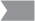# Currently Tested Essential Elements for Mathematics

The documents below detail the currently tested Essential Elements.  For a list of all Dynamic Learning Maps® Essential Elements,, see Essential Elements for Mathematics (pdf).

NOTE: Essential Elements marked with a gray flagare not assessed in Year-End states.

Does my state use the IE or YE model?

## Key to Map Codes

 IP Initial Precursor DP Distal Precursor PP Proximal Precursor T Target S Successor SP Supporting UN Untested

## Guide to EE Codes

 A-CED Creating Equations A-REI Algebra – Reasoning with Equations and Inequalities A-SSE Algebra – Seeing Structure in Expressions EE Expressions and Equations F Functions F-BF Functions – Building Functions F-IF Functions – Interpreting Functions F-LE Functions – Linear, Quadratic, and Exponential Models G Geometry G-CO Geometry – Congruence G-GPE Geometry – Expressing Geometric Properties with Equations G-MG Geometry – Modeling with Geometry MD Measurement and Data NBT Number and Operations in Base Ten N-CN Number and Quantity – The Complex Number System NF Number and Operations – Fractions N-RN Number and Quantity – The Real Number System N-Q Number and Quantity NS The Number System OA Operations and Algebraic Thinking RP Ratios and Proportional Relationships S-CP Statistics and Probability – Conditional Probability and the Rules of Probability S-ID Statistics and Probability – Interpreting Categorical and Quantitative Data S-IC Statistics and Probability – Making Inferences and Justifying Conclusions SP Statistics and Probability